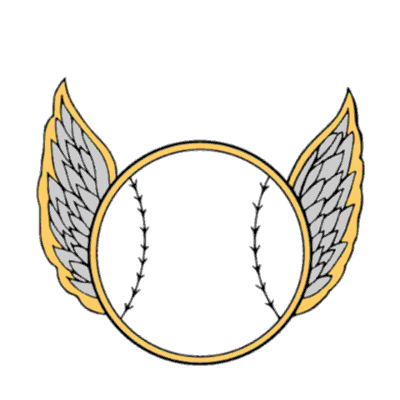# Copying a Point Shape@ from a SearchCursor to a InsertCursor

2216
7
04-11-2018 01:31 PMbyMVP Esteemed Contributor

I am trying to copy a Point from a SearchCursor to a InsertCursor but I can never get the geometry to work.  In past projects I ended up giving up on it and just did a Append then insert to get around it but I want to figure it out (unless this is a good way?).

First note that the Search FC and the Insert FC are different projections.  It may be that arc will not handle this for me.

This is like what I am trying (mini version) but it gives a AttributeError: __exit__

FROM

fields = [ 'SHAPE@']
with arcpy.da.SearchCursor(plotLayer, fields) as cursor:
for row in cursor:

saveShape = row

TO

insertFields = [ 'SHAPE@']

with arcpy.InsertCursor(terraFC, insertFields) as inCursor:
inCursor.insertRow(saveShape)

I have also tried SHAPE@XY and still no.  It seems like I have found code like this that works for Polygons but not points. Almost all examples of Point always use a x,y.

Thanks a lot for any help

Tags (1)
1 Solution

Accepted SolutionsbyMVP Esteemed Contributor

Fortunately, ArcPy Data Access search cursors do projection on the fly, which saves you from having to explicitly project the data after retrieving it.  Also, since you are working with points, ArcPy Data Access insert cursors will automatically create the PointGeometry objects for you from a tuple representing an X,Y coordinate.

``````fields = ["SHAPE@XY", "field1", "field2"]
wkid = # WKID of destination FC
SR = arcpy.SpatialReference(wkid)

with arcpy.da.SearchCursor(plotLayer, fields, spatial_reference=SR) as cur:
with arcpy.da.InsertCursor(terraFC, fields) as icur:
for row in cur:
icur.insertRow(row)‍‍‍‍‍‍‍‍``````
7 RepliesbyMVP Regular Contributor

I've used something like the following:

``````import arcpy

srcFeature = r'C:\Path\To\geodatabase.gdb\test1'
srcFields = ['SHAPE@X', 'SHAPE@Y', 'MyField'] # x & y plus additional fields as needed
srcSR = 3857 # Web Mercator - change as needed

destFeature = r'C:\Path\To\geodatabase.gdb\test2'
destFields = ['SHAPE@X', 'SHAPE@Y', 'MyField'] # x & y plus additional fields as needed
destSR = 4326 # WGS 1984 (Lat/Lon) - change as needed

whereClause = '1=1' # edit as needed

with arcpy.da.InsertCursor(destFeature, destFields) as destCursor: # destination - insert cursor
with arcpy.da.SearchCursor(srcFeature, srcFields, where_clause=whereClause) as srcCursor: # source - search cursor
for srcRow in srcCursor:
destRow = [None]* len(destFields) # initialize destRow for number of fields to be inserted
ptGeometry = arcpy.PointGeometry(arcpy.Point(srcRow, srcRow), arcpy.SpatialReference(srcSR)).projectAs(arcpy.SpatialReference(destSR))
destRow = ptGeometry.firstPoint.X  # x coord
destRow = ptGeometry.firstPoint.Y  # y coord
destRow = srcRow # additional field(s)
destCursor.insertRow(destRow)

del destCursor, srcCursor‍‍‍‍‍‍‍‍‍‍‍‍‍‍‍‍‍‍‍‍‍‍‍``````

For spatial reference, use WKID number.  You can add additional fields, if you want them transferred.  I've added a generic where clause which can be modified if you only have a specific group of points to transfer.  Hope this helps.byMVP Honored Contributor

I'll just draw attention to the fact that in the (correct) example above PointGeometry and Point are two completely different objects, and they must be handled with care.byMVP Esteemed Contributor

Fortunately, ArcPy Data Access search cursors do projection on the fly, which saves you from having to explicitly project the data after retrieving it.  Also, since you are working with points, ArcPy Data Access insert cursors will automatically create the PointGeometry objects for you from a tuple representing an X,Y coordinate.

``````fields = ["SHAPE@XY", "field1", "field2"]
wkid = # WKID of destination FC
SR = arcpy.SpatialReference(wkid)

with arcpy.da.SearchCursor(plotLayer, fields, spatial_reference=SR) as cur:
with arcpy.da.InsertCursor(terraFC, fields) as icur:
for row in cur:
icur.insertRow(row)‍‍‍‍‍‍‍‍``````byMVP Esteemed Contributor

Thanks a lot Joshua - great code.  It took a minute because of a different issue.  If you look close at my post I had the old Insert and missed the new .da part!  Duh.  That fixed a lot.

I did change your code slightly to ask the outgoing FC its projection and then use that.  This way it will work even if the SR is different that the hardcoded wkid.  Works but if this is an issue let me know.

sr = arcpy.Describe(terraFC).spatialReference
fields = ["SHAPE@XY"]
whereClause = "PlotKey = '" + plot + "'"   # only want completed plots from above
with arcpy.da.SearchCursor(plotLayer, fields, whereClause, spatial_reference=sr) as cursor:
for row in cursor:
dataValues.append(row)

for eachData in allDataValues:
with arcpy.da.InsertCursor(terraFC, insertFields) as inCursor:
inCursor.insertRow(eachData)

I also wonder if you did  fields = ["SHAPE@"] if it would work for any geometry type?

It all works!  Thanks again to everyone.byMVP Legendary Contributor

The difference is SHAPE@ returns a geometry object, SHAPE@XY goes an extra step and returns the coordinates.  It depends whether you need to do something with the geometry or the coordinates, a subtle but important distinction

``````for row in arcpy.da.SearchCursor(in_fc, ["SHAPE@"]):
# Print x,y coordinates of each point feature
#
print(row)

<geoprocessing describe geometry object object at 0x000002898716CDA0>
<geoprocessing describe geometry object object at 0x000002898716C6C0>
<geoprocessing describe geometry object object at 0x000002898716CDA0>
... snip

for row in arcpy.da.SearchCursor(in_fc, ["SHAPE@XY"]):
# Print x,y coordinates of each point feature
#
print(row)

(340500.00000000047, 5020500.000000002)
(341500.0000000014, 5020500.000000002)
(342500.00000000093, 5020500.000000002)
(343500.00000000047, 5020500.000000002)
(344500.0, 5020500.000000002)
(340500.00000000047, 5021500.0)
(341500.0000000014, 5021500.0)
(342500.00000000093, 5021500.0)
(343500.00000000047, 5021500.0)
(344500.0, 5021500.0)
(340500.0000000004, 5022500.000000001)
(341500.00000000146, 5022500.000000001)
(342166.66666666855, 5022500.000000001)
(343500.00000000047, 5022500.000000001)
(344500.0, 5022500.000000001)

...snip‍‍‍‍‍‍‍‍‍‍‍‍‍‍‍‍‍‍‍‍‍‍‍‍‍‍‍‍‍‍‍‍‍‍‍``````byMVP Esteemed Contributor

I just want to copy the geometry from A to B.  Not change it in any way.

thanksbyMVP Esteemed Contributor

I tested and using just SHAPE@ works just fine.  That means this code should work for any type of FC.

Thanks